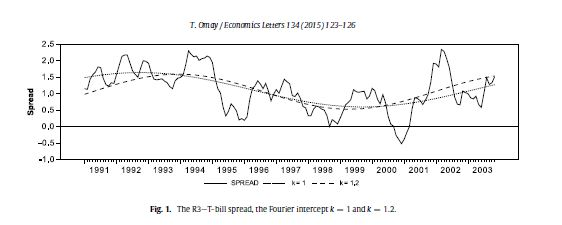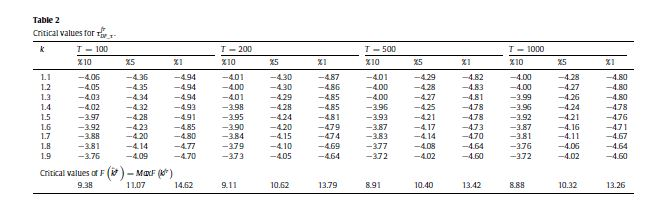### 1. Introduction

In the recent literature, multiple smooth breaks have been modeled by Flexible Fourier Transforms by Becker et al. (2006), Enders and Lee (2012a,b), and Rodrigues and Taylor (2012). The advantages of the Fourier approach include being able to capture the behavior of a deterministic function of unknown form even if the function itself is not periodic, working better than dummy variable methods irrespective of whether the breaks are instantaneous or smooth, and avoiding the problems of selecting the dates, number and form of breaks (Becker et al., 2006, Enders and Lee, 2012a,b; Rodrigues and Taylor, 2012). All these papers pointed out that the single frequency component of the Fourier Transforms should be used for structural break determination; otherwise, the over-filtration problem arises. However, none of them seems toconsider the Fractional Frequency version of their test in their studies.

The Fractional Frequency Flexible Fourier Form (FFFFF) is used in Becker et al. (2004) for the structural break test, namely Trig-test. They attempt to prove that their methodology is better than the conventionally used break tests. Moreover, in their study Becker et al. (2004) show that the best fitting frequency for the US inflation rate between the period 1947:1 to 2011:11 is f * = 1.178, which is fractionally determined when testing for structural breaks. Therefore, by combining the methodologies of Becker et al. (2004) and Enders and Lee (2012b, Henceforth, EL test), this study aims to improve the unit root testing with Fourier Transforms.

### 2. Unit Root Test with FFFFF

The following Fourier de-trending first take place in the FFFFF-ADF testing procedure:

$y_{t}=\beta+\beta_{2}\sin (2\pi k^f t/T)+\beta_{3}\cos (2\pi k t/T)+\epsilon_{t}$

In the second step classical ADF test without any deterministic component employed.

$\Delta\epsilon_{t}=\alpha_{1}\epsilon_{t-1}+\nu_{t}$
Finally, Omay(2015) has used the Davies (1987) methodlogy in order to select the appropirate frequency selection:

De- Trended by FFFFF and IFFFFCritical Values for FFFFFSee Omay (2015) for details

Citation of the Omay(2015) in Google Scholar